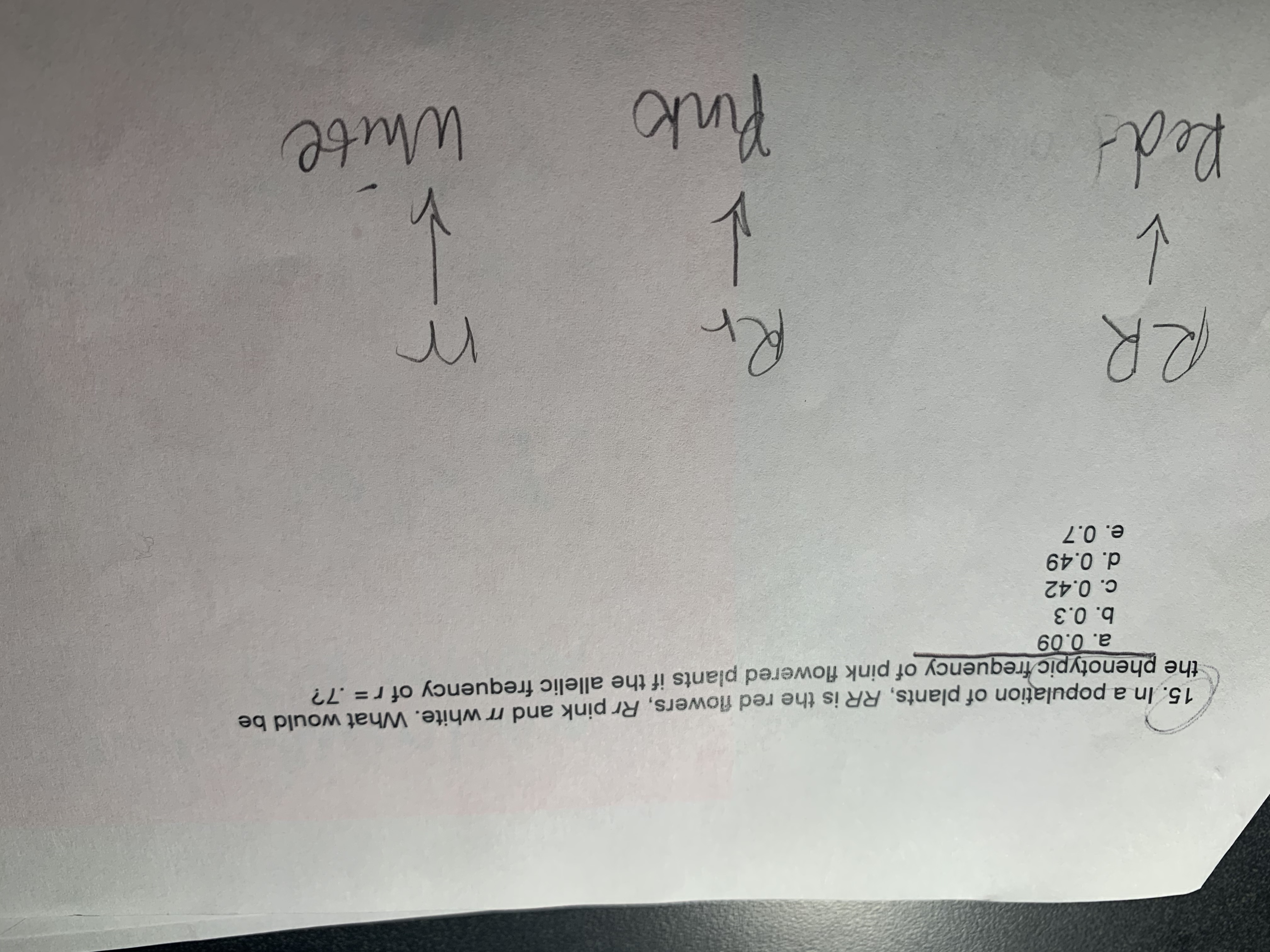# -315. In a population of plants, RR is the red flowers, Rr pink and rr white. What would bethe phenotypic frequency of pink flowered plants if the allelic frequency of r= .7?a. 0.09b. 0.3c. 0.42d. 0.49e. 0.7RR1PinkoWhite

Question
26 views

Im having a hard time figuring out how to calculate this using the allele frequency. I know that r+R= 1 but which would make R=.3 but that is not the answer. How fo I calculate this?help_outlineImage Transcriptionclose-3 15. In a population of plants, RR is the red flowers, Rr pink and rr white. What would be the phenotypic frequency of pink flowered plants if the allelic frequency of r= .7? a. 0.09 b. 0.3 c. 0.42 d. 0.49 e. 0.7 RR 1 Pinko White fullscreen
check_circle

Step 1

In this given population, the single locus allelic frequency is given as r = 0.7. Therefore according to Hardy Weinburg principle allelic frequency of R = 0.3.

In order to determine...

### Want to see the full answer?

See Solution

#### Want to see this answer and more?

Solutions are written by subject experts who are available 24/7. Questions are typically answered within 1 hour.*

See Solution
*Response times may vary by subject and question.
Tagged in

### Biology